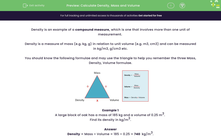# Calculate Density, Mass and Volume

In this worksheet, students will calculate densities, masses and volumes.Key stage:  KS 3

Curriculum topic:   Geometry and Measures

Curriculum subtopic:   Derive and Apply Formulae

Difficulty level:#### Worksheet Overview

Density is an example of a compound measure, which is one that involves more than one unit of measurement.

Density is a measure of mass (e.g. kg, g) in relation to unit volume (e.g. m3, cm3,) and can be measured in kg/m3, g/cm3, etc.

You should know the following formulae and may find the triangle helpful for remembering the three mass, density and volume formulae.Example 1

A large block of oak has a mass of 185 kg and a volume of 0.25 m3

Find its density in kg/m3.

Density = Mass ÷ Volume = 185 ÷ 0.25 = 740  kg/m3.

Example 2

Aluminium has a density of 2.712 g/cm3.  Find the mass of a piece of aluminium with a volume of 12 cm3.

Mass = Density x Volume

Mass = 2.712 x 12 = 32.5 g

Now let's apply these formulae by tackling some questions together!

### What is EdPlace?

We're your National Curriculum aligned online education content provider helping each child succeed in English, maths and science from year 1 to GCSE. With an EdPlace account you’ll be able to track and measure progress, helping each child achieve their best. We build confidence and attainment by personalising each child’s learning at a level that suits them.

Get started